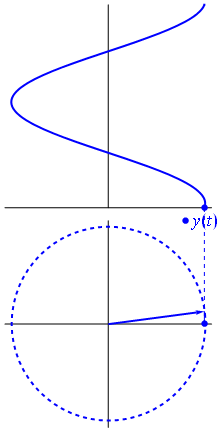# Phasor

Engine of time.

from Wikipedia

http://en.wikipedia.org/wiki/PhasorTraveling along the outer edge of the circle, the distance goes from 0 to 2*PI radians, then starts over again. In degrees it would be 0 to 360. A clock goes from 0 to 12. In Max and Pd the phasor~ objects are normalized to run from 0.0 to 1.0. Think of flattening out the edge of the circle to a straight line.

The phasor as input to a sine or cosine function generates one  cycle of a wave for each revolution – as shown in the above animation. For example, cosine would go from 0 to 1 to 0 to -1 to 0.

If you graph the phasor value, it looks like a sawtooth wave – rising in a ramp from 0.0 to 1.0 then falling straight down to 0.0 to start again.

With waves, the distance around the circle represents time (or phase), the projected cosine value represents amplitude.

##### In science…

“A phasor can be considered a vector rotating about the origin in a complex plane. The cosine function is the projection of the vector onto the real axis. Its amplitude is the modulus of the vector, and its argument is the total phase$\omega t+\theta$. The phase constant$\theta$represents the angle that the vector forms with the real axis at t = 0.”

-WIkipedia

##### In art…

“And the seasons they go round and round
And the painted ponies go up and down
We’re captive on the carousel of time
We can’t return we can only look
Behind from where we came
And go round and round and round
In the circle game.”

-Joni Mitchell

(These lyrics happen at about 4:40 in the video…)